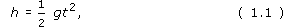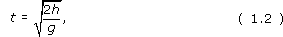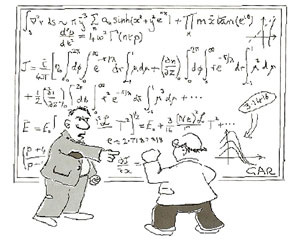Science, Maths & Technology

### Become an OU studentThe restless Universe

Start this free course now. Just create an account and sign in. Enrol and complete the course for a free statement of participation or digital badge if available.

# 2.1.2 Mathematics and quantification

Roger Bacon once said 'Mathematics is the door and the key to the sciences'. This statement aptly summarises the role of mathematics in science, particularly in physics, and it is not hard to see why.

Much of physics is concerned with things that can be measured and quantified, that is, expressed as numbers, multiplied by an appropriate unit of measurement such as a metre or a second. It is natural to turn to mathematics to try to reveal patterns underlying such measured data. This is more than a matter of arithmetic. By Roger Bacon's time the basic ideas of algebra had been developed, mainly by Arabic mathematicians and astronomers. The idea of representing a quantity by a symbol, such as x or t is extremely powerful because it allows us to express general relationships in a very compact way. For example, in the equationthe symbol h represents the height fallen by an object that has been dropped from rest, the symbol t represents the time the object has been falling, and g is a constant with a known value (g = 9.81 metres per second per second). Equation 1.1 encapsulates a wealth of information about falling objects, information that is precise and useful. The tools of algebra allow us to go further. For example, the above equation can be rearranged to readso now, if we know the height fallen by an object, we can work out how long it has taken to fall.

Mathematics provides a natural medium for rational argument. Given an equation that relates various quantities, the rules of mathematics allow that equation to be re-expressed in a number of different but logically equivalent ways, all of which are valid if the original equation was valid. Given two equations, mathematical reasoning allows them to be combined to produce new equations which are again valid if the original equations were valid. Long chains of reasoning can be put together in this way, all of which are guaranteed to be correct provided that the starting points are correct and no mathematical rules are transgressed. Quite often these arguments are so long and detailed that it would be impossible to follow them in ordinary language, even if it were possible to express them at all.

Mathematics has been an immensely effective part of the scientist's toolkit throughout history. It was the increased use of mathematics in the sixteenth and seventeenth centuries, in the hands of individuals such as Galileo Galilei (1564-1642) and Isaac Newton (1642-1727), that opened a new era of physics and marked one of the greatest flowerings of science. Galileo and Newton, it should be noted, were both, at key times in their careers, professors of mathematics. In both cases they brought mathematical precision and rigour to the study of science, and in Newton's case made major breakthroughs in mathematics in the process. The types of mathematics used in physics are extremely varied. Practically every branch of mathematics that has developed over the centuries has been used within physics. Sometimes physics has provided direct inspiration for new mathematical concepts, sometimes abstract mathematical theories have found completely unexpected uses in physics, years after their introduction as products of pure thought.

Despite its power, physics students often find the extensive use of mathematics troublesome and some think of mathematics as providing a barrier to understanding. Do not let this happen to you. From the outset, you should regard mathematics as a friend rather than a foe. As the course progresses, you may meet some mathematical ideas that are new to you, or you may need to improve your ability to use methods you have met before. These are not distractions from trying to understand physics, but are the tools needed to make that understanding possible. It is only through using mathematics that a secure understanding can be achieved. When you see an equation, welcome its concision and clarity and try to 'read' the equation just as you would the large number of words it replaces. Learn to get beneath the squiggles and the equals sign and to understand the quantitative assertion that is being made.Figure 4 “I see through your squiggles.”

Later, you will see how graphs can be used to visualise an equation and how consideration of special cases and trends can help unpack its meaning.

## Question 1

When Jesuits first visited China they spoke about the 'laws of science'. The Chinese thought this was a ridiculous notion: people could be persuaded to obey the laws of the Emperor, but sticks and stones have no intelligence so it is absurd to think of them as 'obeying laws'. How would you respond to this?